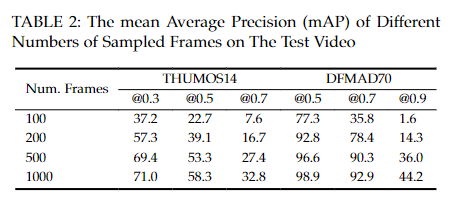Typeset Tabulars and Arrays with LATEX3.

• Github repo
• Manual (2022A)
• The one called in the Overleaf may not be the latest version, depending on the texlive version used.

# An example

\documentclass[10pt,journal,compsoc]{IEEEtran}
\usepackage{tabularray}

\begin{table}[!t]
\caption{Comparison of Attention mechanisms}
\label{D70_compare}
\centering
\begin{tblr}{
colspec = {l c | X[c]},
hline{1, 6} = {1.5pt, solid},
hline{2} = {1-2}{1pt, dashed},
hline{3},
row{5} = {cyan8},
}
\SetCell[c=2]{c} two column & & \SetCell[r=2]{c} two rows &\\
attention & out. proj. &\\
space-time & sth1 & 90.3\\
space-time & sth2 & 70.6\\
space-time & sth3 & 90.3
\end{tblr}
\end{table}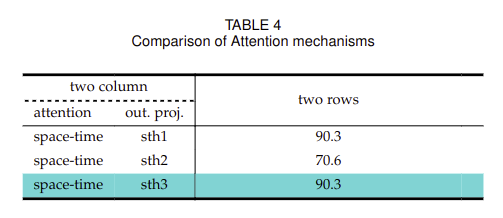# Mandatory argument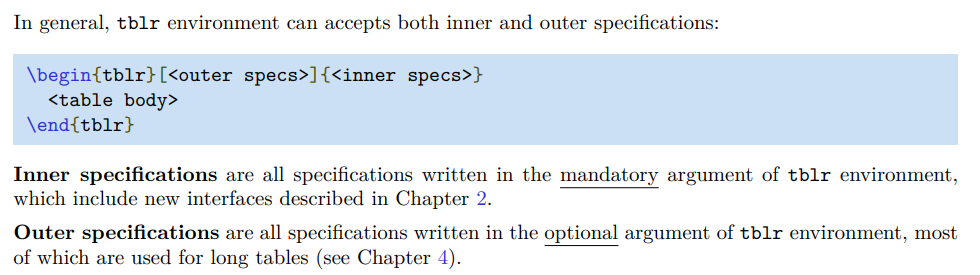BASIC Inner Specifications in mandatory argument (the column of new interfaces):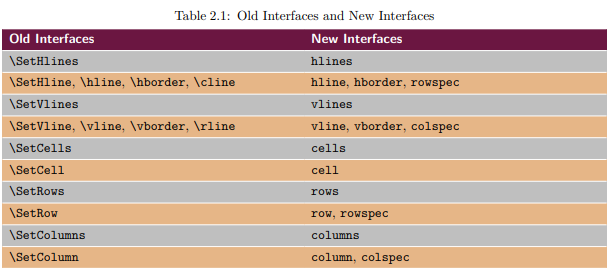We categorize the basic keys into three classes: Cells, Lines, and Rows and columns, which are discussed in the next three sections, and then the last section we introduce the extra keys.

## The Old and New interfaces

The Old interfaces and New interfaces are two styles for the same function.

The Old interfaces (command-style):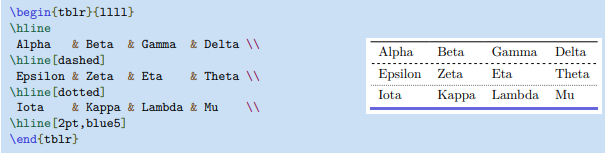The New interfaces (Innter specifications-sytle, recommended):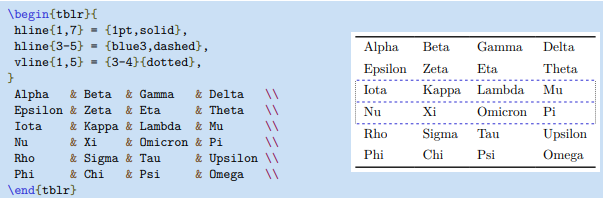## rows and columns.

rows = {<kwargs_of_row>}, colmuns = {<kwargs_of_column>}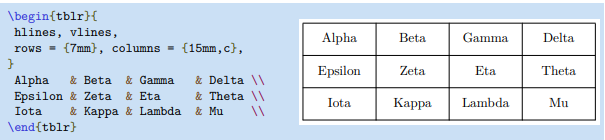## row and column

row{<row_index>} = {<kwargs_of_row>}, column{<column_index>} = {<kwargs_of_column>}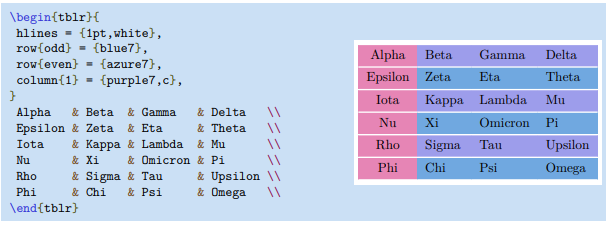You may select multiple rows/column indexes at once, e.g., row{1,3,9}, row{3-6}, row{even}, row{odd}.

## List of keys (kwargs) for row and column: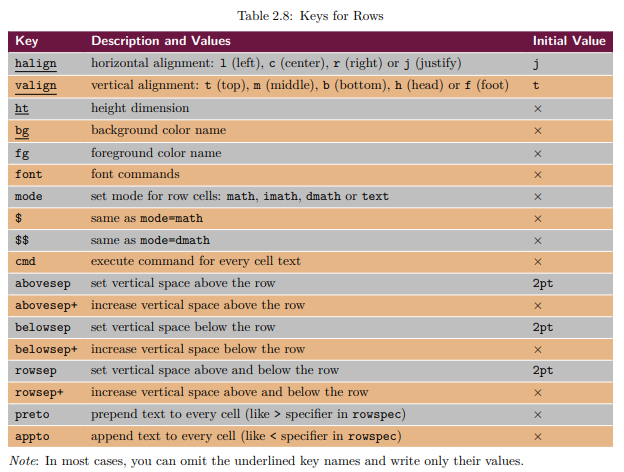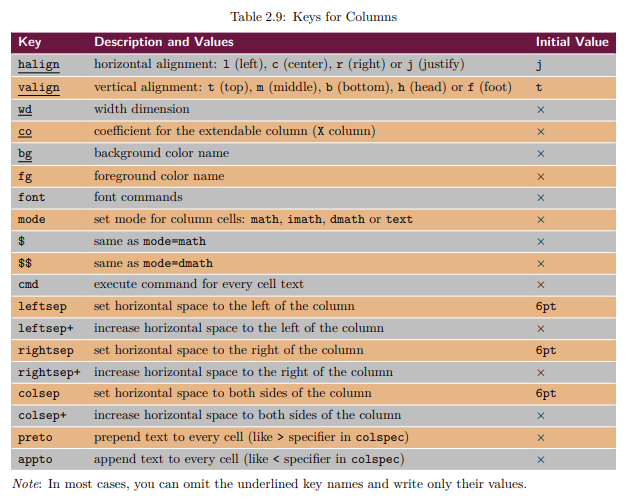You can find many examples demonstrating the usage of these keys in the manul.

## colspec and rowspec

colspec={<list_of_column_types>}, rowspec={<list_of_row_types>}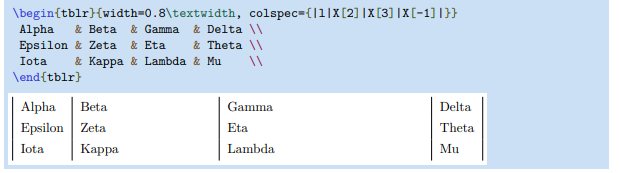The \begin{tblr}{|X|X|X|} is equal to \begin{tblr}{colspec={|X|X|X|}}. The colspec= can be omitted if it is the only key inside the mandatory argument.

### Column types X

Here I only introduce the X column type. Please refer to the manul for more column types and row types.

X[<width>, <alignment>]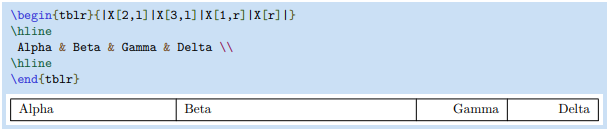<width>=-1 means the width depends on the text length.

## cells

cells = {<kwargs_of_cell>}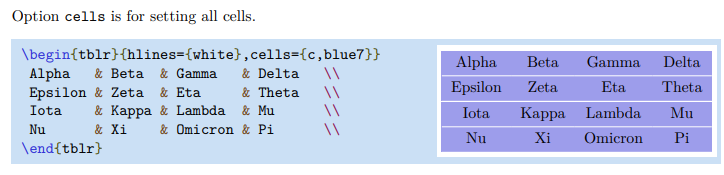## cell{i}{j}

cell{i}{j} = {<kwargs_of_span>}{<kwargs_of_cell>}, the <kwargs_of_span> is about the multiple rows and columns and can be ommited.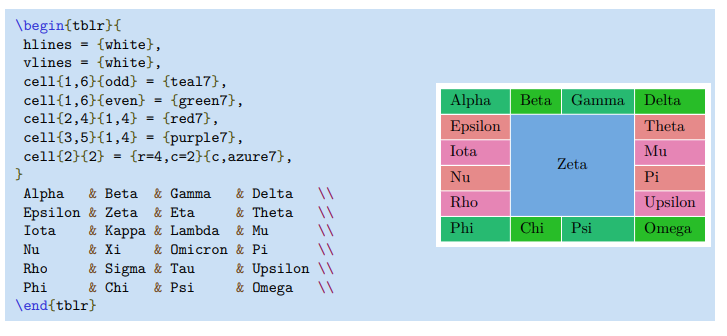You may use the OLD interface \SetCell for building cells: \SetCell[<kwargs_of_span>]{<kwargs_of_cell>} Cell content (note the difference between the [] and {})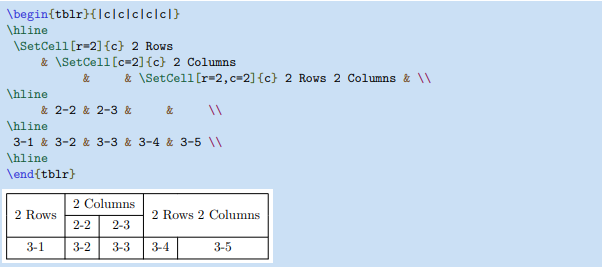## List of keys for cell: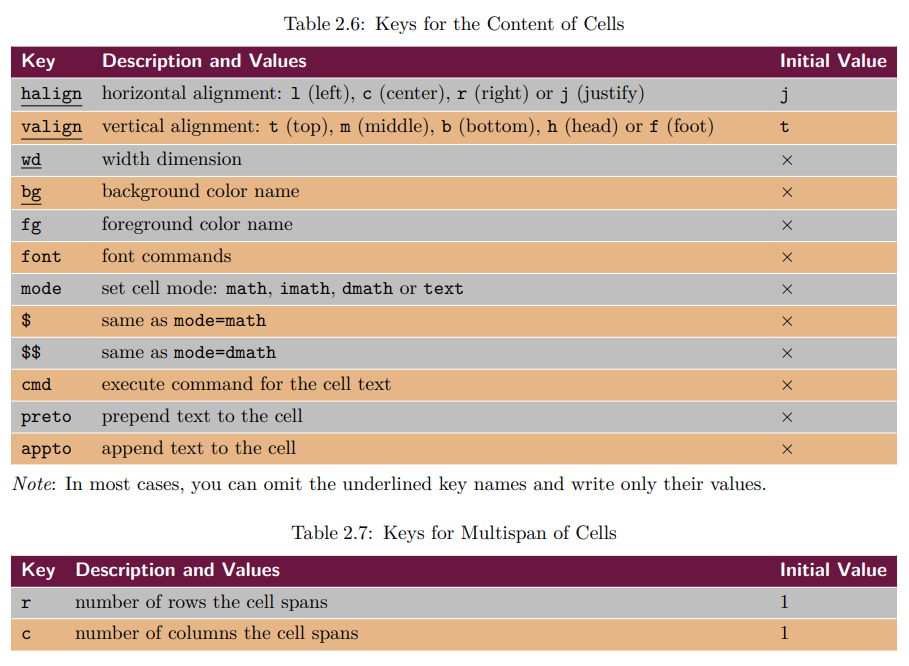## hlines and vlines

hlines = {<index_of_line>}{<index_of_column>}{<kwargs_of_line>}, where the <index_of_line> is normally omitted and only specified when multiple adjacent lines is needed, the <index_of_column> can be omitted and its default values {-} means drawing hlines across all columns, the <kwargs_of_line> can also be omitted meaning the default line format. vlines is the same with hlines but specify the index of rows to for the vertical lines to cross.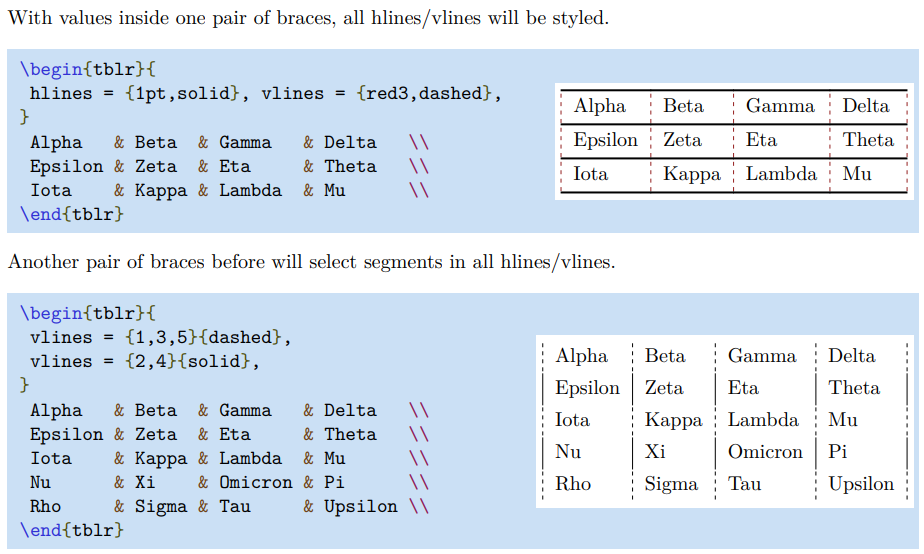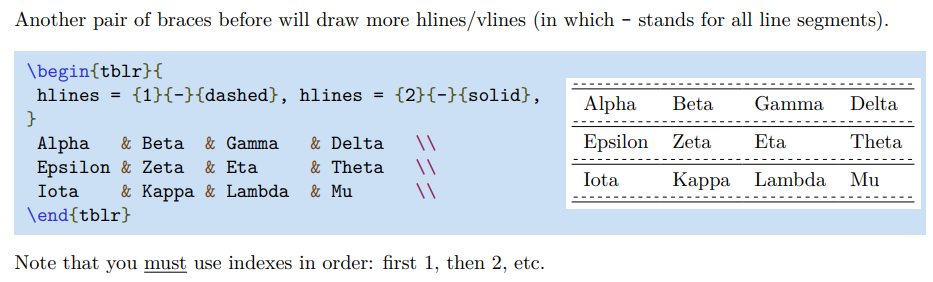## hline and vlines

hline{<index_of_row>} = <index_of_line>}{<index_of_column>}{<kwargs_of_line>}, share the same arguments with hlines but can specify which row by <index_of_row> to draw horizontal lines, vice versa for the vline.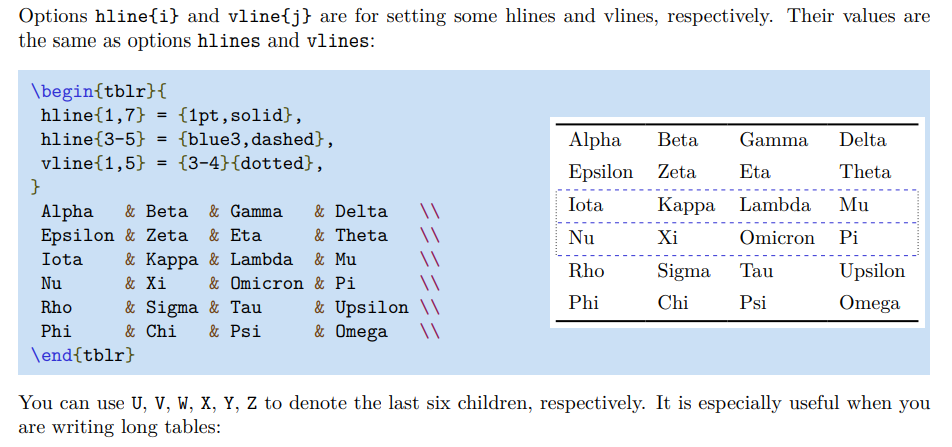Similar to the cell, you may use the OLD interfaces \SetHline,\hline, and \cline for building horizontal lines: \SetHline = [<index_of_line>]{<index_of_column>}{<kwargs_of_line>} \hline = [<kwargs_of_line>] \cline = [<kwargs_of_line>]{<index_of_column>}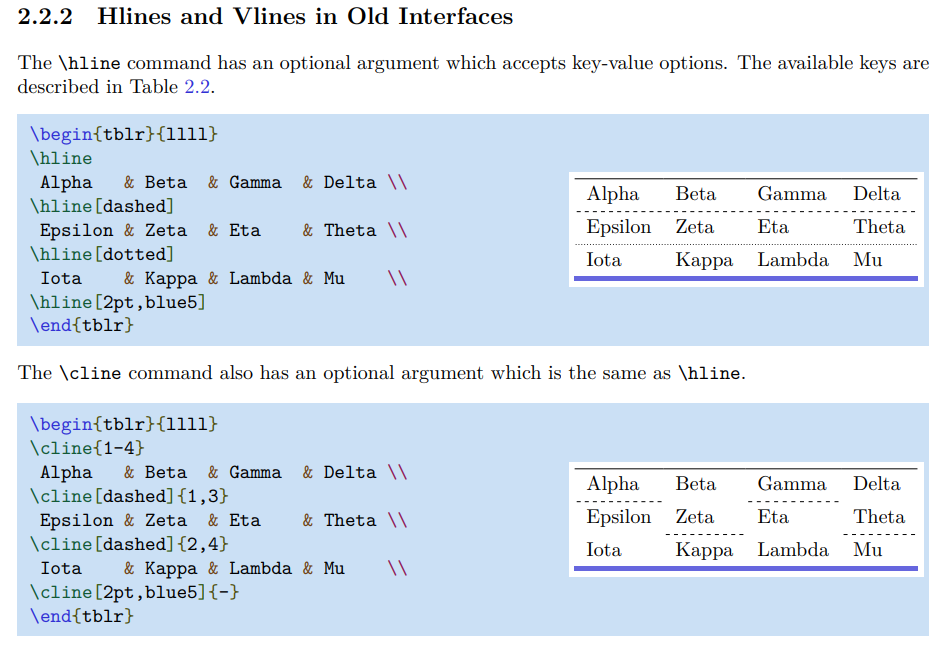The usage of SetVline, \vline, and \rline is similar to the above ones.

## List of keys for hline and vline: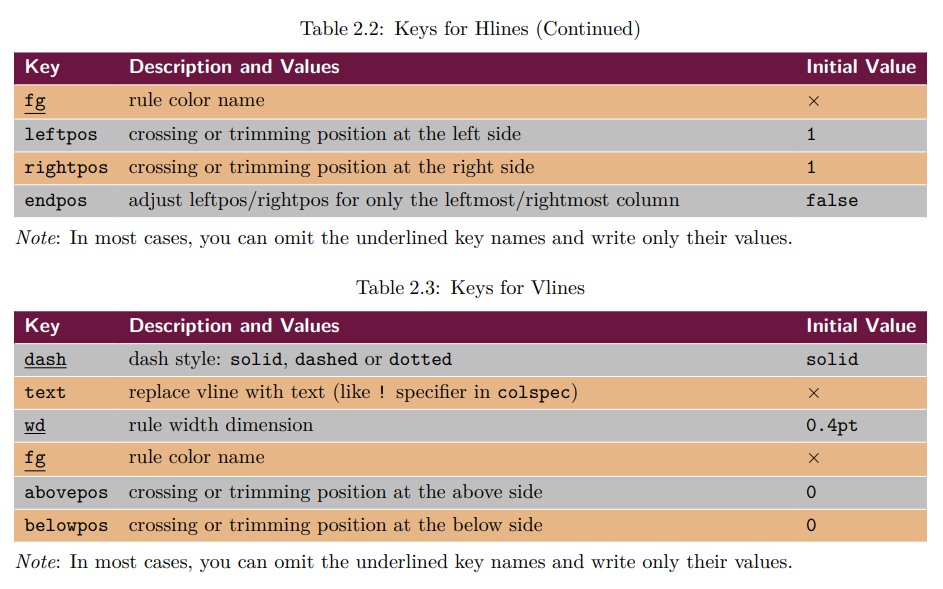# Others

Other arguments that can be used in the inner specification in the tblr: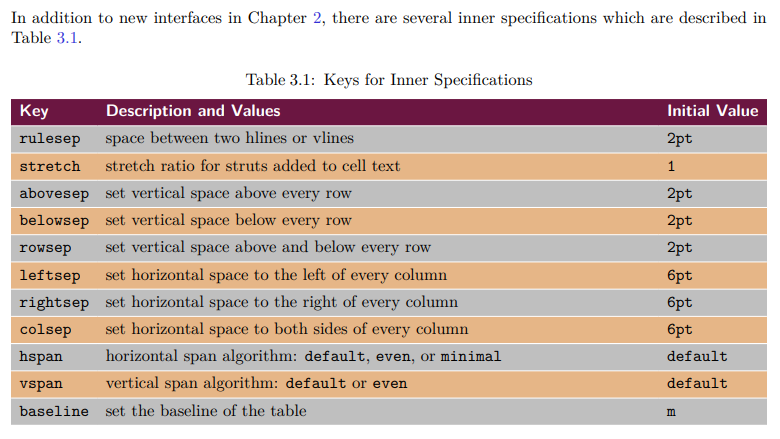There is another argument call width seems was missed in the table. You can use it like width=0.8\textwidth.

# Miscellaneous

## Using talltblr in the table environment

talltblr is designed to contain caption, therefore directly putting talltblr inside the table environment will cause two captions:

\renewcommand\TblrOverlap{#1}

\begin{document}
\begin{table*}[!tb]
\caption{Comparison with State-of-the-Art Methods on THUMOS14.}
\label{T14_compare}
\centering
\begin{talltblr}[
caption = {Some introduction. $\dag$: the model with focal loss},
note{$\dag$} = {the model with focal loss},
]{
colspec = {c X},
hlines,
vlines,
}
G-TAD$^\dag$  & Foo \\
BMN\TblrNote{$\dag$}  & Bar\\
\end{talltblr}
\end{table*}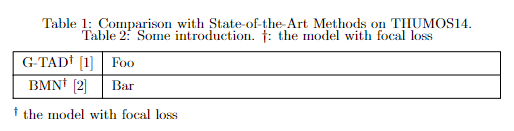One simple solution is that we do NOT use it inside the table but a solely talltblr. While this may cause inconsistency if your other tables are of type table. For example, the distance between the caption and the table (headsep) and the size of caption maybe different for the table and talltblr.

Another tricky solution is to keep the talltblr inside the table but delete the caption of talltable:

\renewcommand\TblrOverlap{#1}

\begin{document}
\begin{table*}[!tb]
\caption{Comparison with State-of-the-Art Methods on THUMOS14.}
\label{T14_compare}
\centering
%%%%%%%%%%%%%%%%%%%%
\begin{talltblr}[
caption = {Some introduction. $\dag$: the model with focal loss},
note{$\dag$} = {the model with focal loss},
]{
colspec = {c X},
hlines,
vlines,
}
G-TAD$^\dag$  & Foo \\
BMN\TblrNote{$\dag$}  & Bar\\
\end{talltblr}
\end{table*}

\begin{table*}[!tb]
\caption{Comparison with State-of-the-Art Methods on THUMOS14.}
\label{T14_compare}
\begin{tabularx}{1\textwidth}{|c|X|}
\hline
G-TAD$^\dag$  & Foo \\
\hline
BMN\TblrNote{$\dag$}  & Bar\\
\hline
\end{tabularx}
\end{table*}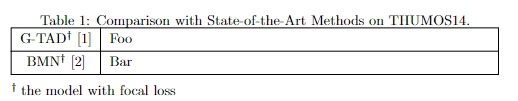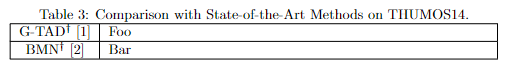Notes: Not applied to longtblr but only talltblr. If applying on the longtblr, the white space after detelting the header still remain.

## rowsep and stretch

Default (rowsep=2pt, stretch=1):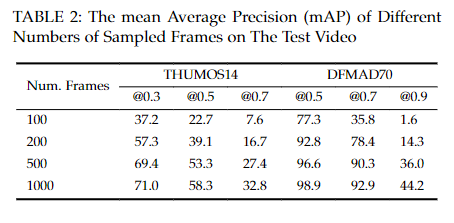rowsep=1pt: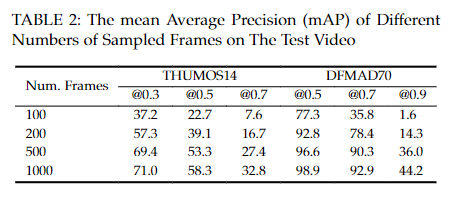stretch=0.7: